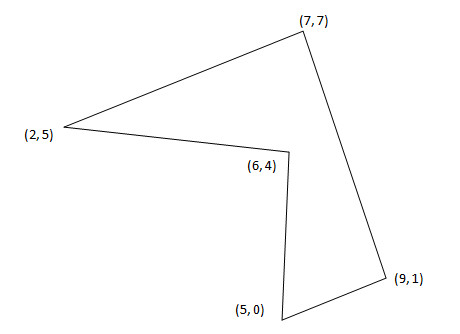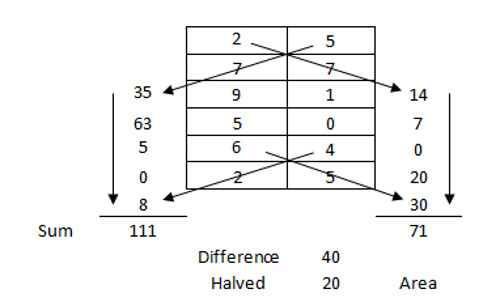# Area MattersIf you know the vertices of a polygon, here’s an interesting way to find its area:

1. Arrange the vertices in a vertical list, repeating the first vertex at the end (see below).
2. Multiply diagonally downward both ways as shown.
3. Add the products on each side.
4. Find the difference of these sums.
5. Halve that difference to get the area.This works for any polygon, no matter the number of points, so long as it doesn’t intersect itself. It’s a slight restatement of the shoelace formula.

(Thanks, Derek, Dan, and Kyle.)